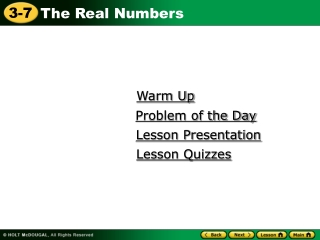DownloadDownload PresentationWarm Up

Warm Up

Download PresentationWarm Up

- - - - - - - - - - - - - - - - - - - - - - - - - - - E N D - - - - - - - - - - - - - - - - - - - - - - - - - - -
Presentation Transcript

1. Warm Up Problem of the Day Lesson Presentation Lesson Quizzes

2. 1. 119 2. – 15 3. 2 4. – 123 Warm Up Each square root is between two integers. Name the two integers. Use a calculator to find each value. Round to the nearest tenth. 10 and 11 –4 and –3 1.4 –11.1

3. Problem of the Day The circumference of a circle is approximately 3.14 times its diameter. A circular path 1 meter wide has an inner diameter of 100 meters. How much farther is it around the outer edge of the path than the inner edge? 6.28 m

4. Learn to determine if a number is rational or irrational.

5. Vocabulary irrational number real number Density Property

6. Biologists classify animals based on shared characteristics. The cardinal is an animal, a vertebrate, a bird, and a passerine. Animals You already know that some numbers can be classified as whole numbers, integers, or rational numbers. Vertebrates Birds Passerines

7. Recall that rational numbers can be written as fractions. Rational numbers can also be written as decimals that either terminate or repeat. 4 5 23 3 = 3.8 = 0.6 1.44 = 1.2

8. 2 ≈1.4142135623730950488016… Caution! A repeating decimal may not appear to repeat on a calculator, because calculators show a finite number of digits. Irrational numberscan only be written as decimals that do not terminate or repeat. If a whole number is not a perfect square, then its square root is an irrational number.

9. The set of real numbers consists of the set of rational numbers and the set of irrational numbers.

10. 16 2 4 2 = = 2 Additional Example 1: Classifying Real Numbers Write all names that apply to each number. A. 5 is a whole number that is not a perfect square. 5 irrational, real B. –12.75 –12.75 is a terminating decimal. rational, real 16 2 C. whole, integer, rational, real

11. 9 = 3 81 3 9 3 = = 3 Check It Out: Example 1 Write all names that apply to each number. 9 A. whole, integer, rational, real –35.9 –35.9 is a terminating decimal. B. rational, real 81 3 C. whole, integer, rational, real

12. 0 3 = 0 Additional Example 2: Determining the Classification of All Numbers State if each number is rational, irrational, or not a real number. A. 21 irrational 0 3 B. rational

13. 2 3 2 3 4 9 = Additional Example 2: Determining the Classification of All Numbers State if each number is rational, irrational, or not a real number. C. –4 not a real number 4 9 D. rational

14. Check It Out: Example 2 State if each number is rational, irrational, or not a real number. A. 23 is a whole number that is not a perfect square. 23 irrational 9 0 B. undefined, so not a real number

15. 8 9 8 9 64 81 = Check It Out: Example 2 State if each number is rational, irrational, or not a real number. C. –7 not a real number 64 81 D. rational

16. The Density Property of real numbers states that between any two real numbers is another real number. This property is also true for rational numbers, but not for whole numbers or integers. For instance, there is no integer between –2 and –3.

17. 2 5 3 5 Find a real number between 3 and 3 . 2 5 3 5 5 5 1 2 3 + 3 ÷ 2 = 6 ÷ 2 = 7 ÷ 2 = 3 4 5 1 5 2 5 3 5 3 3 3 3 3 4 1 2 3 3 5 2 5 1 2 A real number between 3 and 3is 3 . Additional Example 3: Applying the Density Property of Real Numbers There are many solutions. One solution is halfway between the two numbers. To find it, add the numbers and divide by 2.

18. 3 7 4 7 Find a real number between 4 and 4 . 7 7 1 2 3 7 4 7 = 9 ÷ 2 = 4 4 + 4 ÷ 2 = 8 ÷ 2 5 7 1 7 6 7 2 7 3 7 4 7 4 4 4 4 4 4 1 2 4 4 7 1 2 3 7 A real number between 4 and 4 is 4 . Check It Out: Example 3 There are many solutions. One solution is halfway between the two numbers. To find it, add the numbers and divide by 2.

19. Lesson Quizzes Standard Lesson Quiz Lesson Quiz for Student Response Systems

20. 4 • 9 3 4 3 8 Find a real number between –2 and –2 . 5 8 Possible answer –2 . Lesson Quiz Write all names that apply to each number. 16 2 2. – 1. 2 real, irrational real, integer, rational State if each number is rational, irrational, or not a real number. 25 0 4. 3. rational not a real number 5.

21. Lesson Quiz for Student Response Systems 1. Identify all names that apply to . A. real, irrational B. real, rationalC.not real, irrational D.not real, rational

22. Lesson Quiz for Student Response Systems 2. Identify the name that applies to . A. irrational B. rationalC.not a real number D.none

23. Lesson Quiz for Student Response Systems 3. Identify a real number between . A. –4 B. C. D.